# Infinitesimal facts for kids

Kids Encyclopedia Facts

Infinitesimals are quantities that are too small to measure.

## Infinitesimal calculus

Newton and Leibniz developed the calculus based on an intuitive notion of an infinitesimal. In 1870, Karl Weierstraß provided the first rigorous treatment of the calculus, using the limit method. But in 1960, Abraham Robinson found that infinitesimals also provide a rigorous basis for the calculus. Many calculus courses still begin with the difficult limit concept, but others now adopt the easier to understand infinitesimal concept.

In differential calculus, in both the limit and infinitesimal versions, the velocity of a particle is represented by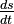$\tfrac{ds}{dt}$, where s is the position of the particle and t the time at which the velocity is measured. In infinitesimal calculus, ds and dt are simply very small quantities. In general, the term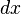$dx$ is called the differential of the variable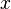$x$.

Assume that s = t2, we have that at time t + dt, s + ds = (t + dt)2. From these equations, we can determine ds in terms of dt by using simple algebra as follows: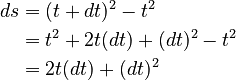\begin{align} ds & = (t + dt)^2-t^2 \\ & = t^2 + 2t(dt) + (dt)^2-t^2 \\ & = 2t(dt) + (dt)^2 \end{align} which means that: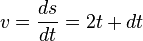$v = \frac{ds}{dt} = 2t + dt$

Because dt is infinitesimal, one can just ignore it, so the instantanous velocity is v = 2t. One gets the same result using the limit version, with greater rigour. The infinitesimal derivation can also be made more rigorous.

## Images for kidsInfinitesimal Facts for Kids. Kiddle Encyclopedia.